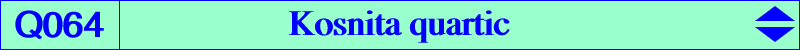too complicated to be written here. Click on the link to download a text file.X(3), X(4), X(186), X(2574), X(2575) anti-points, see Table 77 isogonal conjugates of the Ix-anticevian points, see Table 23The Kosnita quartic Q064 is a circular inversible focal nodal quartic. The singular focus is X(186), the inverse of H in the circumcircle. The node is O with nodal tangents parallel to the asymptotes of the Jerabek hyperbola. Q064 has two real asymptotes also parallel to the asymptotes of the Jerabek hyperbola and meeting the Euler line at X(2072). Q064 meets the circumcircle at the same points as the Orthocubic K006. The tangents at these points concur at O. The circle with diameter the two other points on the sideline BC is orthogonal to the polar circle. Similarly for CA and AB. Q064 is very similar to Q023. The third polar of O is the nodal cubic K389, analogous to the Lemoine cubic K009. See also CL037. Locus properties Q064 is the locus of P such that the circumcevian triangle of P is orthologic to the Kosnita triangle (together with the circumcircle). See Table 31. Obviously, the Kosnita triangle can be replaced by any homothetic triangle such as the orthic, tangential, extangents triangles for example. Let M be a point, M* its isogonal conjugate, M' its inverse in the circumcircle. The points M*, M' and H are collinear if and only if M lies on Q064. See Q019 for an analogous quartic when H is replaced by K.